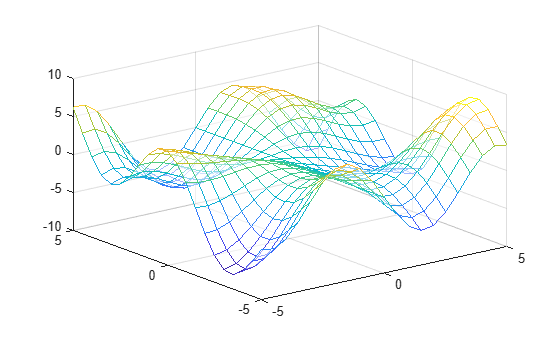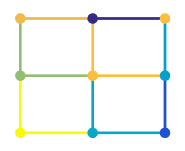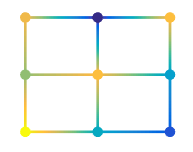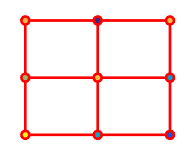# mesh

Mesh surface plot

## Syntax

``mesh(X,Y,Z)``
``mesh(Z)``
``mesh(Z,C)``
``mesh(___,C)``
``mesh(ax,___)``
``mesh(___,Name,Value)``
``s = mesh(___)``

## Description

example

````mesh(X,Y,Z)` creates a mesh plot, which is a three-dimensional surface that has solid edge colors and no face colors. The function plots the values in matrix `Z` as heights above a grid in the x-y plane defined by `X` and `Y`. The edge colors vary according to the heights specified by `Z`.```
````mesh(Z)` creates a mesh plot and uses the column and row indices of the elements in `Z` as the x- and y-coordinates.```
````mesh(Z,C)` additionally specifies the color of the edges.```

example

````mesh(___,C)` additionally specifies the color of the edges.```
````mesh(ax,___)` plots into the axes specified by `ax` instead of the current axes. Specify the axes as the first input argument.```

example

````mesh(___,Name,Value)` specifies surface properties using one or more name-value pair arguments. For example, `'FaceAlpha',0.5` creates a semitransparent mesh plot.```

example

````s = mesh(___)` returns the chart surface object. Use `s` to modify the mesh plot after it is created. For a list of properties, see Surface Properties.```

## Examples

collapse all

Create three matrices of the same size. Then plot them as a mesh plot. The plot uses `Z` for both height and color.

```[X,Y] = meshgrid(-8:.5:8); R = sqrt(X.^2 + Y.^2) + eps; Z = sin(R)./R; mesh(X,Y,Z)```Specify the colors for a mesh plot by including a fourth matrix input, `C`. The mesh plot uses `Z` for height and `C` for color. Specify the colors using a colormap, which uses single numbers to stand for colors on a spectrum. When you use a colormap, `C` is the same size as `Z`. Add a color bar to the graph to show how the data values in `C` correspond to the colors in the colormap.

```[X,Y] = meshgrid(-8:.5:8); R = sqrt(X.^2 + Y.^2) + eps; Z = sin(R)./R; C = X.*Y; mesh(X,Y,Z,C) colorbar```Specify the colors for a mesh plot by including a fourth matrix input, `CO`. The mesh plot uses `Z` for height and `CO` for color. Specify the colors using truecolor, which uses triplets of numbers to stand for all possible colors. When you use truecolor, if `Z` is `m`-by-`n`, then `CO` is `m`-by-`n`-by-3. The first page of the array indicates the red component for each color, the second page indicates the green component, and the third page indicates the blue component.

```[X,Y,Z] = peaks(25); CO(:,:,1) = zeros(25); % red CO(:,:,2) = ones(25).*linspace(0.5,0.6,25); % green CO(:,:,3) = ones(25).*linspace(0,1,25); % blue mesh(X,Y,Z,CO)```Create a semitransparent mesh surface by specifying the `FaceAlpha` name-value pair with `0.5` as the value. To allow further modifications, assign the surface object to the variable `s`.

```[X,Y] = meshgrid(-5:.5:5); Z = Y.*sin(X) - X.*cos(Y); s = mesh(X,Y,Z,'FaceAlpha','0.5')``````s = Surface with properties: EdgeColor: 'flat' LineStyle: '-' FaceColor: [1 1 1] FaceLighting: 'none' FaceAlpha: 0.5000 XData: [21x21 double] YData: [21x21 double] ZData: [21x21 double] CData: [21x21 double] Show all properties ```

Use `s` to access and modify properties of the mesh plot after it is created. For example, add color to the face of the mesh plot by setting the `FaceColor` property.

`s.FaceColor = 'flat';`## Input Arguments

collapse all

x-coordinates, specified as a matrix the same size as `Z`, or as a vector with length `n`, where `[m,n] = size(Z)`. If you do not specify values for `X` and `Y`, `mesh` uses the vectors `(1:n)` and `(1:m)`.

You can use the `meshgrid` function to create `X` and `Y` matrices.

The `XData` property of the surface object stores the x-coordinates.

Example: `X = 1:10`

Example: `X = [1 2 3; 1 2 3; 1 2 3]`

Example: `[X,Y] = meshgrid(-5:0.5:5)`

Data Types: `single` | `double` | `int8` | `int16` | `int32` | `int64` | `uint8` | `uint16` | `uint32` | `uint64` | `categorical` | `datetime` | `duration`

y-coordinates, specified as a matrix the same size as `Z` or as a vector with length `m`, where `[m,n] = size(Z)`. If you do not specify values for `X` and `Y`, `mesh` uses the vectors `(1:n)` and `(1:m)`.

You can use the `meshgrid` function to create the `X` and `Y` matrices.

The `YData` property of the surface object stores the y-coordinates.

Example: `Y = 1:10`

Example: `Y = [1 1 1; 2 2 2; 3 3 3]`

Example: `[X,Y] = meshgrid(-5:0.5:5)`

Data Types: `single` | `double` | `int8` | `int16` | `int32` | `int64` | `uint8` | `uint16` | `uint32` | `uint64` | `categorical` | `datetime` | `duration`

z-coordinates, specified as a matrix. `Z` must have at least two rows and two columns.

`Z` specifies the height of the mesh plot at each x-y coordinate. If you do not specify the colors, then `Z` also specifies the mesh edge colors.

The `ZData` property of the surface object stores the z-coordinates.

Example: `Z = [1 2 3; 4 5 6]`

Example: `Z = sin(x) + cos(y)`

Data Types: `single` | `double` | `int8` | `int16` | `int32` | `int64` | `uint8` | `uint16` | `uint32` | `uint64` | `categorical` | `datetime` | `duration`

Color array, specified as an `m`-by-`n` matrix of colormap indices or as an `m`-by-`n`-by-`3` array of RGB triplets, where `Z` is `m`-by-`n`.

• To use colormap colors, specify `C` as a matrix. For each grid point on the mesh surface, `C` indicates a color in the colormap. The `CDataMapping` property of the surface object controls how the values in `C` correspond to colors in the colormap.

• To use truecolor colors, specify `C` as an array of RGB triplets.

The `CData` property of the surface object stores the color array. For additional control over the surface coloring, use the `FaceColor` and `EdgeColor` properties.

Axes to plot in, specified as an `axes` object. If you do not specify the axes, then `mesh` plots into the current axes.

### Name-Value Pair Arguments

Specify optional comma-separated pairs of `Name,Value` arguments. `Name` is the argument name and `Value` is the corresponding value. `Name` must appear inside quotes. You can specify several name and value pair arguments in any order as `Name1,Value1,...,NameN,ValueN`.

Example: `mesh(X,Y,Z,'FaceAlpha',0.5)` creates a semitransparent mesh plot.

### Note

The properties listed here are only a subset. For a full list, see Surface Properties.

Edge line color, specified as one of the values listed here. The default color of `[0 0 0]` corresponds to black edges.

ValueDescription
`'none'`Do not draw the edges.
`'flat'`

Use a different color for each edge based on the values in the `CData` property. First you must specify the `CData` property as a matrix the same size as `ZData`. The color value at the first vertex of each face (in the positive x and y directions) determines the color for the adjacent edges. You cannot use this value when the `EdgeAlpha` property is set to `'interp'`.`'interp'`

Use interpolated coloring for each edge based on the values in the `CData` property. First you must specify the `CData` property as a matrix the same size as `ZData`. The color varies across each edge by linearly interpolating the color values at the vertices. You cannot use this value when the `EdgeAlpha` property is set to `'flat'`.RGB triplet, hexadecimal color code, or color name

Use the specified color for all the edges. This option does not use the color values in the `CData` property.RGB triplets and hexadecimal color codes are useful for specifying custom colors.

• An RGB triplet is a three-element row vector whose elements specify the intensities of the red, green, and blue components of the color. The intensities must be in the range `[0,1]`; for example,``` [0.4 0.6 0.7]```.

• A hexadecimal color code is a character vector or a string scalar that starts with a hash symbol (`#`) followed by three or six hexadecimal digits, which can range from `0` to `F`. The values are not case sensitive. Thus, the color codes `'#FF8800'`, `'#ff8800'`, `'#F80'`, and `'#f80'` are equivalent.

Alternatively, you can specify some common colors by name. This table lists the named color options, the equivalent RGB triplets, and hexadecimal color codes.

Color NameShort NameRGB TripletHexadecimal Color CodeAppearance
`'red'``'r'``[1 0 0]``'#FF0000'``'green'``'g'``[0 1 0]``'#00FF00'``'blue'``'b'``[0 0 1]``'#0000FF'``'cyan'` `'c'``[0 1 1]``'#00FFFF'``'magenta'``'m'``[1 0 1]``'#FF00FF'``'yellow'``'y'``[1 1 0]``'#FFFF00'``'black'``'k'``[0 0 0]``'#000000'``'white'``'w'``[1 1 1]``'#FFFFFF'`Here are the RGB triplets and hexadecimal color codes for the default colors MATLAB® uses in many types of plots.

`[0 0.4470 0.7410]``'#0072BD'``[0.8500 0.3250 0.0980]``'#D95319'``[0.9290 0.6940 0.1250]``'#EDB120'``[0.4940 0.1840 0.5560]``'#7E2F8E'``[0.4660 0.6740 0.1880]``'#77AC30'``[0.3010 0.7450 0.9330]``'#4DBEEE'``[0.6350 0.0780 0.1840]``'#A2142F'`Line style, specified as one of the options listed in this table.

Line StyleDescriptionResulting Line
`'-'`Solid line`'--'`Dashed line`':'`Dotted line`'-.'`Dash-dotted line`'none'`No lineNo line

Face color, specified as one of the values in this table.

ValueDescription
`'flat'`

Use a different color for each face based on the values in the `CData` property. First you must specify the `CData` property as a matrix the same size as `ZData`. The color value at the first vertex of each face (in the positive x and y directions) determines the color for the entire face. You cannot use this value when the `FaceAlpha` property is set to `'interp'`.`'interp'`

Use interpolated coloring for each face based on the values in the `CData` property. First you must specify the `CData` property as a matrix the same size as `ZData`. The color varies across each face by interpolating the color values at the vertices. You cannot use this value when the `FaceAlpha` property is set to `'flat'`.RGB triplet, hexadecimal color code, or color name

Use the specified color for all the faces. This option does not use the color values in the `CData` property.`'texturemap'`Transform the color data in `CData` so that it conforms to the surface.
`'none'`Do not draw the faces.

RGB triplets and hexadecimal color codes are useful for specifying custom colors.

• An RGB triplet is a three-element row vector whose elements specify the intensities of the red, green, and blue components of the color. The intensities must be in the range `[0,1]`; for example,``` [0.4 0.6 0.7]```.

• A hexadecimal color code is a character vector or a string scalar that starts with a hash symbol (`#`) followed by three or six hexadecimal digits, which can range from `0` to `F`. The values are not case sensitive. Thus, the color codes `'#FF8800'`, `'#ff8800'`, `'#F80'`, and `'#f80'` are equivalent.

Alternatively, you can specify some common colors by name. This table lists the named color options, the equivalent RGB triplets, and hexadecimal color codes.

Color NameShort NameRGB TripletHexadecimal Color CodeAppearance
`'red'``'r'``[1 0 0]``'#FF0000'``'green'``'g'``[0 1 0]``'#00FF00'``'blue'``'b'``[0 0 1]``'#0000FF'``'cyan'` `'c'``[0 1 1]``'#00FFFF'``'magenta'``'m'``[1 0 1]``'#FF00FF'``'yellow'``'y'``[1 1 0]``'#FFFF00'``'black'``'k'``[0 0 0]``'#000000'``'white'``'w'``[1 1 1]``'#FFFFFF'`Here are the RGB triplets and hexadecimal color codes for the default colors MATLAB uses in many types of plots.

`[0 0.4470 0.7410]``'#0072BD'``[0.8500 0.3250 0.0980]``'#D95319'``[0.9290 0.6940 0.1250]``'#EDB120'``[0.4940 0.1840 0.5560]``'#7E2F8E'``[0.4660 0.6740 0.1880]``'#77AC30'``[0.3010 0.7450 0.9330]``'#4DBEEE'``[0.6350 0.0780 0.1840]``'#A2142F'`Face transparency, specified as one of these values:

• Scalar in range `[0,1]` — Use uniform transparency across all the faces. A value of `1` is fully opaque and `0` is completely transparent. Values between `0` and `1` are semitransparent. This option does not use the transparency values in the `AlphaData` property.

• `'flat'` — Use a different transparency for each face based on the values in the `AlphaData` property. The transparency value at the first vertex determines the transparency for the entire face. First you must specify the `AlphaData` property as a matrix the same size as the `ZData` property. The `FaceColor` property also must be set to `'flat'`.

• `'interp'` — Use interpolated transparency for each face based on the values in `AlphaData` property. The transparency varies across each face by interpolating the values at the vertices. First you must specify the `AlphaData` property as a matrix the same size as the `ZData` property. The `FaceColor` property also must be set to `'interp'`.

• `'texturemap'` — Transform the data in `AlphaData` so that it conforms to the surface.

Effect of light objects on faces, specified as one of these values:

• `'flat'` — Apply light uniformly across each face. Use this value to view faceted objects.

• `'gouraud'` — Vary the light across the faces. Calculate the light at the vertices and then linearly interpolate the light across the faces. Use this value to view curved surfaces.

• `'none'` — Do not apply light from light objects to the faces.

To add a light object to the axes, use the `light` function.

### Note

The `'phong'` value has been removed. Use `'gouraud'` instead.